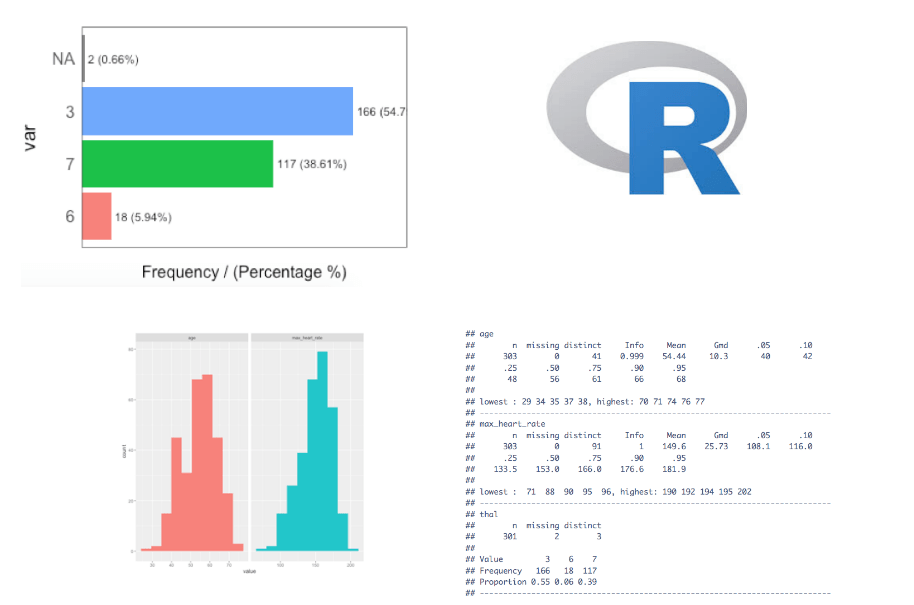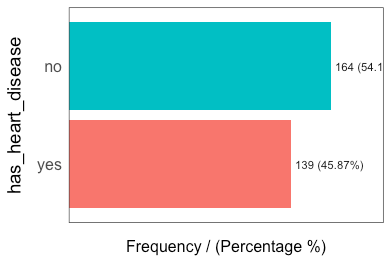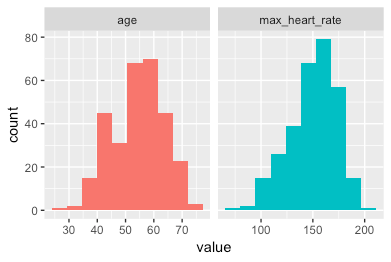[This article was first published on R - Data Science Heroes Blog, and kindly contributed to R-bloggers]. (You can report issue about the content on this page here)
Want to share your content on R-bloggers? click here if you have a blog, or here if you don't.Hi there!

tl;dr: Exploratory data analysis (EDA) the very first step in a data project. We will create a code-template to achieve this with one function.

## Introduction

EDA consists of univariate (1-variable) and bivariate (2-variables) analysis.
In this post we will review some functions that lead us to the analysis of the first case.

• Step 1 – First approach to data
• Step 2 – Analyzing categorical variables
• Step 3 – Analyzing numerical variables
• Step 4 – Analyzing numerical and categorical at the same time

Covering some key points in a basic EDA:

• Data types
• Outliers
• Missing values
• Distributions (numerically and graphically) for both, numerical and categorical variables.

### Type of analysis results

They can be two: informative or operative.

Informative – For example plots, or any long variable summary. We cannot filter data from it, but give us a lot of information at once. Most used on the EDA stage.

Operative – The results can be used to take an action directly on the data workflow (for example, selecting any variables whose percentage of missing values are below 20%). Most used in the Data Preparation stage.

### Setting-up

Uncoment in case you don’t have any of these libraries:

```# install.packages("tidyverse")
# install.packages("funModeling")
# install.packages("Hmisc")
```

A newer version of `funModeling` has been released on Ago-1, please update 😉

```library(funModeling)
library(tidyverse)
library(Hmisc)
```

### tl;dr (code)

Run all the functions in this post in one-shot with the following function:

```basic_eda <- function(data)
{
glimpse(data)
df_status(data)
freq(data)
profiling_num(data)
plot_num(data)
describe(data)
}
```

Replace `data` with your data, and that's it!:

`basic_eda(my_amazing_data)`

Creating the data for this example

Using the `heart_disease` data (from `funModeling` package). We will take only 4 variables for legibility.

```data=heart_disease %>% select(age, max_heart_rate, thal, has_heart_disease)
```

## Step 1 - First approach to data

Number of observations (rows) and variables, and a `head` of the first cases.

```glimpse(data)

## Observations: 303
## Variables: 4
## \$ age               <int> 63, 67, 67, 37, 41, 56, 62, 57, 63, 53, 57, ...
## \$ max_heart_rate    <int> 150, 108, 129, 187, 172, 178, 160, 163, 147,...
## \$ thal              <fct> 6, 3, 7, 3, 3, 3, 3, 3, 7, 7, 6, 3, 6, 7, 7,...
## \$ has_heart_disease <fct> no, yes, yes, no, no, no, yes, no, yes, yes,...
```

Getting the metrics about data types, zeros, infinite numbers, and missing values:

```df_status(data)

##            variable q_zeros p_zeros q_na p_na q_inf p_inf    type unique
## 1               age       0       0    0 0.00     0     0 integer     41
## 2    max_heart_rate       0       0    0 0.00     0     0 integer     91
## 3              thal       0       0    2 0.66     0     0  factor      3
## 4 has_heart_disease       0       0    0 0.00     0     0  factor      2
```

`df_status` returns a table, so it is easy to keep with variables that match certain conditions like:
+ Having at least 80% of non-NA values (`p_na < 20`)
+ Having less than 50 unique values (`unique <= 50`)

? TIPS:

• Are all the variables in the correct data type?
• Variables with lots of zeros or `NA`s?
• Any high cardinality variable?

## Step 2 - Analyzing categorical variables

`freq` function runs for all factor or character variables automatically:

```freq(data)
``````##   thal frequency percentage cumulative_perc
## 1    3       166      54.79              55
## 2    7       117      38.61              93
## 3    6        18       5.94              99
## 4 <NA>         2       0.66             100
``````##   has_heart_disease frequency percentage cumulative_perc
## 1                no       164         54              54
## 2               yes       139         46             100

##  "Variables processed: thal, has_heart_disease"
```

? TIPS:

• If `freq` receives one variable -`freq(data\$variable)`- it retruns a table. Useful to treat high cardinality variables (like zip code).
• Export the plots to jpeg into current directory: `freq(data, path_out = ".")`
• Does all the categories make sense?
• Lots of missing values?
• Always check absolute and relative values

## Step 3 - Analyzing numerical variables

We will see: `plot_num` and `profiling_num`. Both run automatically for all numerical/integer variables:

### Graphically

```plot_num(data)
```Export the plot to jpeg: `plot_num(data, path_out = ".")`

? TIPS:

• Try to identify high-unbalanced variables
• Visually check any variable with outliers

### Quantitatively

`profiling_num` runs for all numerical/integer variables automatically:

```data_prof=profiling_num(data)

##         variable mean std_dev variation_coef p_01 p_05 p_25 p_50 p_75 p_95
## 1            age   54       9           0.17   35   40   48   56   61   68
## 2 max_heart_rate  150      23           0.15   95  108  134  153  166  182
##   p_99 skewness kurtosis iqr        range_98     range_80
## 1   71    -0.21      2.5  13        [35, 71]     [42, 66]
## 2  192    -0.53      2.9  32 [95.02, 191.96] [116, 176.6]
```

? TIPS:

• Try to describe each variable based on its distribution (also useful for reporting)
• Pay attention to variables with high standard deviation.
• Select the metrics that you are most familiar with: `data_prof %>% select(variable, variation_coef, range_98)`: A high value in `variation_coef` may indictate outliers. `range_98` indicates where most of the values are.

## Step 4 - Analyzing numerical and categorical at the same time

`describe` from Hmisc package.

```library(Hmisc)
describe(data)

## data
##
##  4  Variables      303  Observations
## ---------------------------------------------------------------------------
## age
##        n  missing distinct     Info     Mean      Gmd      .05      .10
##      303        0       41    0.999    54.44     10.3       40       42
##      .25      .50      .75      .90      .95
##       48       56       61       66       68
##
## lowest : 29 34 35 37 38, highest: 70 71 74 76 77
## ---------------------------------------------------------------------------
## max_heart_rate
##        n  missing distinct     Info     Mean      Gmd      .05      .10
##      303        0       91        1    149.6    25.73    108.1    116.0
##      .25      .50      .75      .90      .95
##    133.5    153.0    166.0    176.6    181.9
##
## lowest :  71  88  90  95  96, highest: 190 192 194 195 202
## ---------------------------------------------------------------------------
## thal
##        n  missing distinct
##      301        2        3
##
## Value         3    6    7
## Frequency   166   18  117
## Proportion 0.55 0.06 0.39
## ---------------------------------------------------------------------------
## has_heart_disease
##        n  missing distinct
##      303        0        2
##
## Value        no  yes
## Frequency   164  139
## Proportion 0.54 0.46
## ---------------------------------------------------------------------------
```

Really useful to have a quick picture for all the variables. But is not as operative as `freq` and `profiling_num` when we want to use its results to change our data workflow.

? TIPS:

• Check min and max values (outliers)
• Check Distributions (same as before)

PS: Does anyone remember the function that creates a single-page with a data summary? Wanted to mention here...

That's all by now! 🙂

PC.